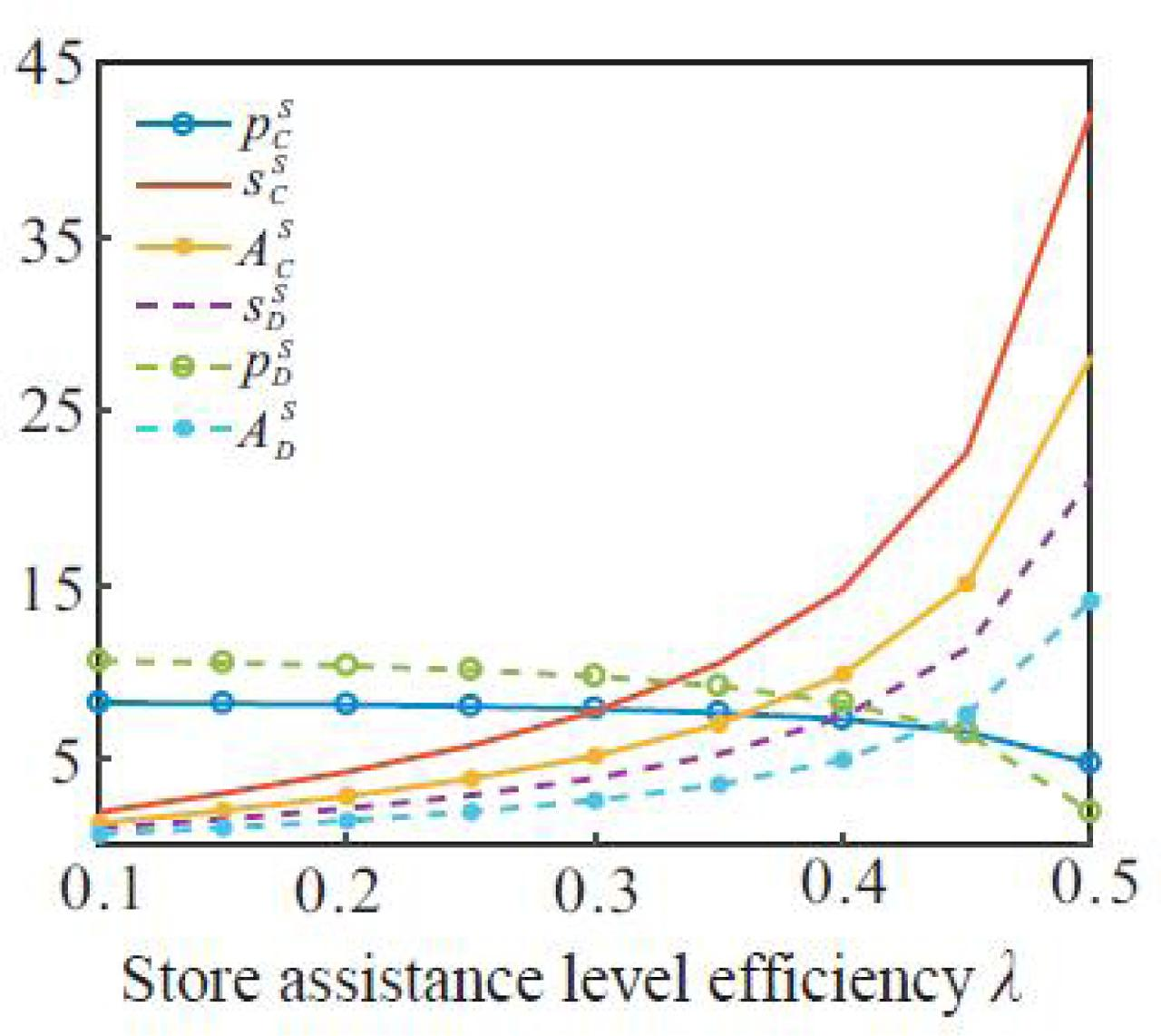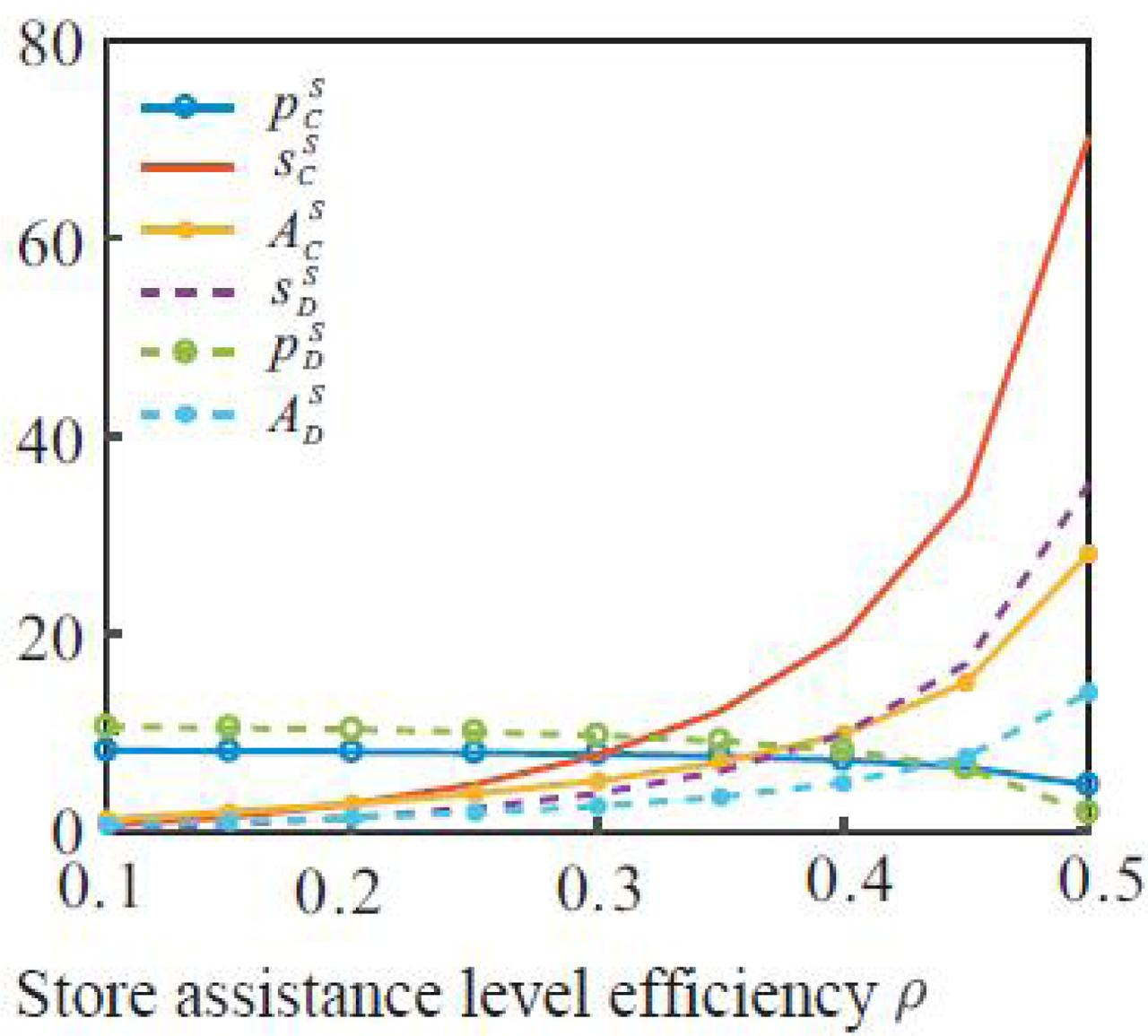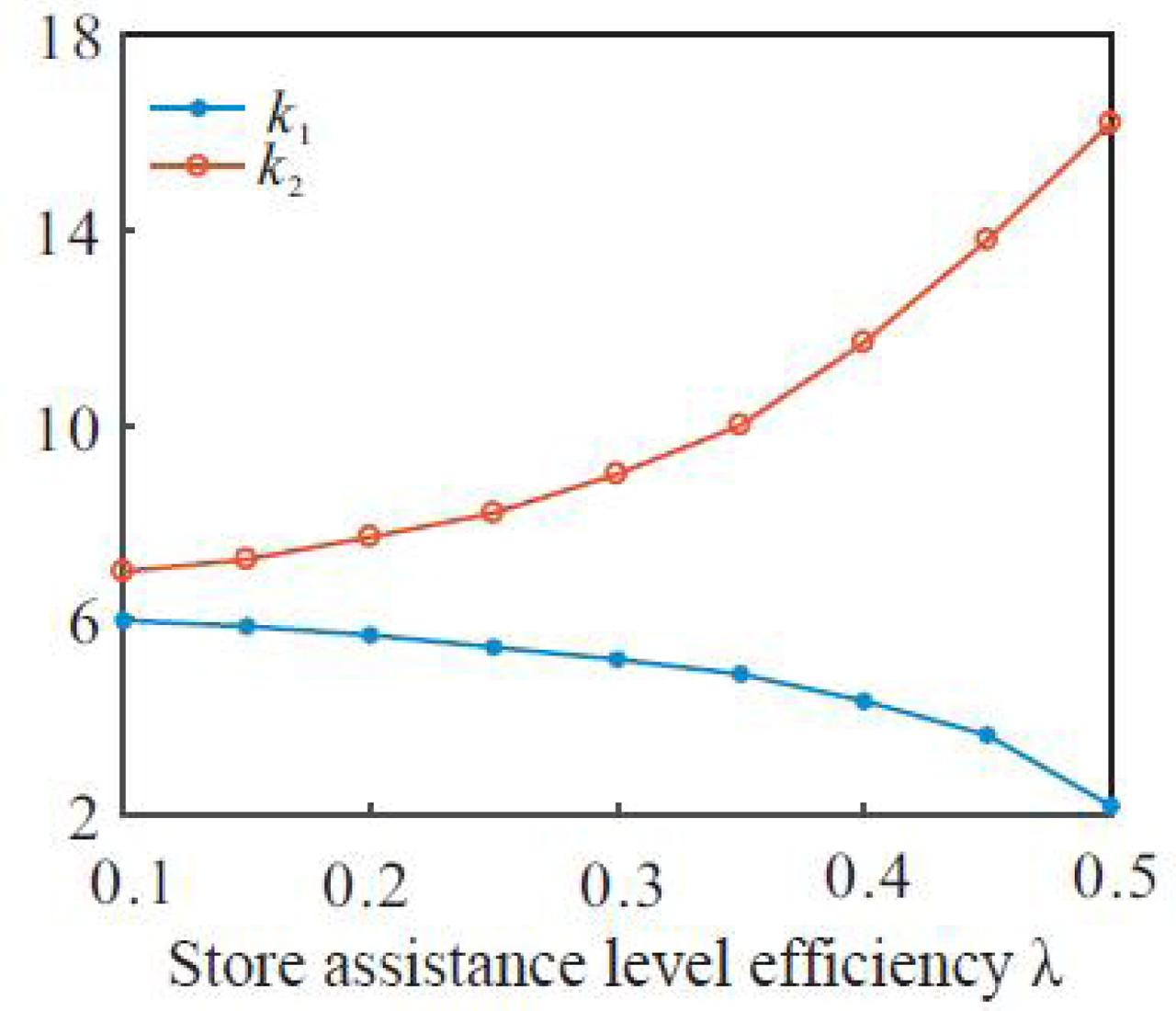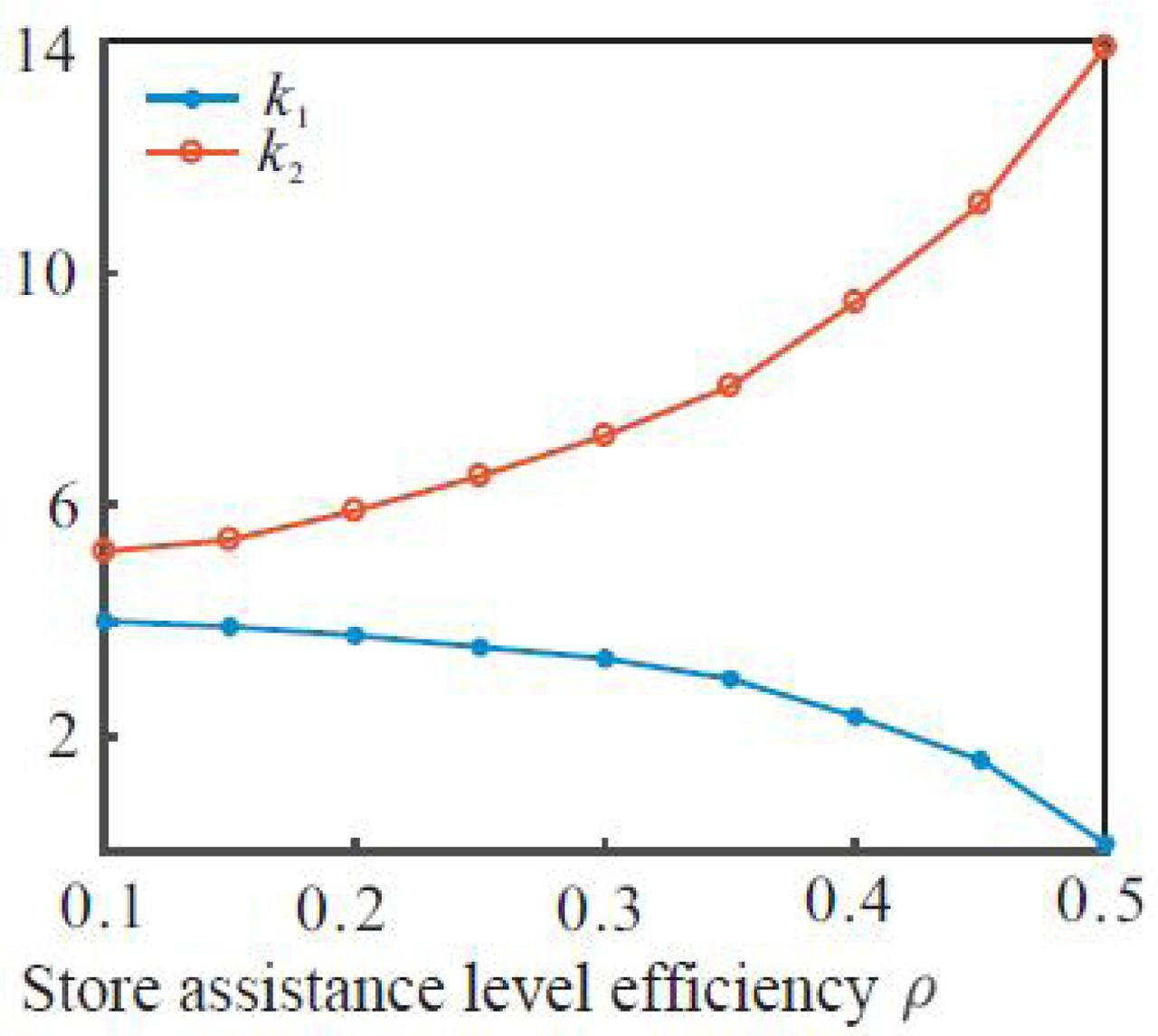Informacje o czasopiśmieFormat
Czasopismo
eISSN
2444-8656
Pierwsze wydanie
01 Jan 2016
Częstotliwość wydawania
2 razy w roku
Języki
AngielskiOtwarty dostęp

# Nonlinear Differential Equations in Cross-border E-commerce Controlling Return Rate

###### Przyjęty: 17 Mar 2022
Informacje o czasopiśmieFormat
Czasopismo
eISSN
2444-8656
Pierwsze wydanie
01 Jan 2016
Częstotliwość wydawania
2 razy w roku
Języki
Angielski

This article constructs a nonlinear differential equation model that incorporates non-defective return factors and minimizes the expected cost of the inventory control problem under the cross-border e-commerce overseas warehouse model. Under the dynamic background, the problem of pricing and shop assistant decision-making in the supply chain is studied, and the differential game model is constructed. We use differential game theory to give pricing and store assistance strategies under decentralized and centralized decision-making. At the same time, we designed a contract to achieve supply chain coordination in this context. Numerical examples calculate the optimal return price and optimal order quantity under supply chain coordination.

#### MSC 2010

Introduction

As market competition continues to intensify, allowing customers to return goods has become a means of competition widely adopted by retailers. For example, JD.com and Tmall allow customers to return unsatisfied products. Returning goods will incur logistics, inspection, and repackaging costs . It is estimated that the US electronics industry spent 13.8 billion US dollars in processing returns in 2007. The main reason for returns is that the products cannot match the needs of customers. Therefore, how to reduce the product return rate is an important issue that retailers face to solve. Current methods to reduce product returns include improving the product design quality, charging replacement fees, and shortening product delivery time. In addition, providing store assistance services can also effectively reduce the product return rate.

Research on customer returns is relatively rich. Some scholars have studied the supply chain decision-making problems affected by returns under different channel structures and gave the chain members' preference for channel structure selection. Some scholars assume that the amount of refunds affects demand and solves the problem of inventory decision-making and return policy formulation in the supply chain. These documents focus on analyzing the impact of customer returns without considering the issue of reduction in return rates. Some scholars believe that store ancillary services can reduce the unreasonable return rate of products and improve the impact of the return rate on market demand. He gave the product pricing and dual-channel strategy of competing companies. Some scholars have further studied the issue of how companies choose to store auxiliary service strategies. The results show that the strength of the difference in the return rate affects the company's strategic choices . Some scholars have constructed a shop assistant decision-making model in the context of asymmetric customer return information. Some scholars have extended the store-assisted decision-making problem to the VMI scenario.

In recent years, certain results have been achieved in studying supply chain optimization and coordination issues affected by returns from a dynamic perspective . By establishing a differential game model and solving the problem, the supply chain's optimal pricing and store-assisted strategy under the background of consignment sales are given. Design a dynamic consignment price contract to achieve supply chain coordination. However, the study did not fully consider the return processing costs caused by product returns and the impact of returns on demand, which is not completely consistent with the actual situation.

This article comprehensively considers the impact of product return rate on market demand. Research on the pricing of supply chain and auxiliary decision-making of stores in a dynamic context. The article uses differential game theory to give pricing and store assistance strategies under decentralized and centralized decision-making. At the same time, a contract is designed to realize supply chain coordination under this background.

Problem description and model establishment

This article considers a supply chain consisting of a manufacturer and a retailer. The manufacturer produces the product and sells the product to the end consumer through the retailer. Retailers allow consumers to return unsatisfactory products after purchasing products . The returned products can be sold again but will incur disposal costs for the retailer. In addition, retailers can reduce the return rate of products by improving store assistance to increase product sales and profits.

Symbol description

cM is the manufacturer's unit production cost. cR is the return disposal cost of the retailer. w(t) is the wholesale price of the product. p(t) is the retail price of the product. A(t) is the store assistance effort invested by the retailer at time t. S(t) is the store assistance level at time t. Q(t) is the product return rate at time t. Ji(i = M, R or C) is the profit function of the manufacturer, retailer or the entire supply chain.

Model assumptions

In the context of the research of this article, the level of store assistance is related to the store assistance efforts invested by retailers. The following differential equation describes it: $S˙(t)=ρA(t)−δS(t)$ \dot S\left( t \right) = \rho A\left( t \right) - \delta S\left( t \right)

S(0) = S0 represents the initial store assistance level. ρ indicates that the store assists efforts to improve the efficiency of service levels. δ represents the attenuation rate of the store assistance level.

The product return rate Q(t) ≥ 0 depends on the level of store assistance. This article is expressed in linear form $Q(t)=Qmax−λS(t)$ Q\left( t \right) = {Q_{\max }} - \lambda S\left( t \right)

Qmax ∈ (0, 1) is a constant, which represents the maximum return rate possible for this type of product. λ > 0 represents the efficiency of the store assistance level in reducing the product return rate. In addition, market demand is affected by both the product price and the return rate, namely D(t) ϕβ p(t) − θQ(t). ϕ represents the market capacity of the product. β represents the impact of retail prices on market demand. θ represents the impact of product return rate on market demand.

Store assistance cost is the quadratic function of store assistance effort, that is, C(A(t)) = kA2(t) / 2, k is the effort cost coefficient . Assume that the supply chain can operate indefinitely and consider that manufacturers and retailers have the same discount rate r > 0. Therefore, the profit functions of manufacturers, retailers and the entire supply chain are respectively $JM=∫0+∞e−rt(w(t)−cM)D(t)dt$ {J_M} = \int_0^{ + \infty } {{e^{ - rt}}\left( {w\left( t \right) - {c_M}} \right)D\left( t \right)dt} $JR=∫0+∞e−rt[(p(t)−w(t)−cRQ(t))D(t)−C(A(t))]dt$ {J_R} = \int_0^{ + \infty } {{e^{ - rt}}\left[ {\left( {p\left( t \right) - w\left( t \right) - {c_R}Q\left( t \right)} \right)D\left( t \right) - C\left( {A\left( t \right)} \right)} \right]dt} $JC=∫0+∞e−rt[(p(t)−cM−cRQ(t))D(t)−C(A(t))]dt$ {J_C} = \int_0^{ + \infty } {{e^{ - rt}}\left[ {\left( {p\left( t \right) - {c_M} - {c_R}Q\left( t \right)} \right)D\left( t \right) - C\left( {A\left( t \right)} \right)} \right]dt}

Centralized decision making

Under centralized decision-making, all members of the supply chain decide the price of the product and the auxiliary strategy of the store, namely decision p(t) and A(t). $pC*(t)=−λ(βcR−θ)2β(S0−SC)e−rCt+pCS$ p_C^*\left( t \right) = - {{\lambda \left( {\beta {c_R} - \theta } \right)} \over {2\beta }}\left( {{S_0} - {S^C}} \right){e^{ - {r_C}t}} + p_C^S $AC*(t)=−βk(r+2δ)−ψC2βkρ(S0−SCS)e−rCt+ACS$ A_C^*\left( t \right) = - {{\beta k\left( {r + 2\delta } \right) - \psi C} \over {2\beta k\rho }}\left( {{S_0} - S_C^S} \right){e^{ - {r_C}t}} + A_C^S

The optimal path for the store assistance level is $SC*(t)=(S0−SCS)e−rCt+SCS$ S_C^*\left( t \right) = \left( {{S_0} - S_C^S} \right){e^{ - {r_C}t}} + S_C^S

In $ψC=βk[βk(r+2δ)2−2ρ2λ2(βcR+θ)2],rC=ψC−βkr2βk,SCS=λρ2(βcR+θ)(ϕ−βcM−(βcR+θ)Qmax)2βkδ(r+δ)−ρ2λ2(βcR+θ)2pCS=ϕ+βcM+(βcR−θ)Qmax2β−λ(βcR−θ)2βSCSACS=λδρ(βcR−θ)(ϕ−βcM−(βcR+θ)Qmax)2βkδ(r+δ)−ρ2λ2(βcR+θ)2$ \matrix{ {{\psi _C} = \sqrt {\beta k\left[ {\beta k{{\left( {r + 2\delta } \right)}^2} - 2{\rho ^2}{\lambda ^2}{{\left( {\beta {c_R} + \theta } \right)}^2}} \right]} ,} \hfill \cr {{r_C} = {{{\psi _C} - \beta kr} \over {2\beta k}},} \hfill \cr {S_C^S = {{\lambda {\rho ^2}\left( {\beta {c_R} + \theta } \right)\left( {\phi - \beta {c_M} - \left( {\beta {c_R} + \theta } \right){Q_{\max }}} \right)} \over {2\beta k\delta \left( {r + \delta } \right) - {\rho ^2}{\lambda ^2}{{\left( {\beta {c_R} + \theta } \right)}^2}}}} \hfill \cr {p_C^S = {{\phi + \beta {c_M} + \left( {\beta {c_R} - \theta } \right){Q_{\max }}} \over {2\beta }} - {{\lambda \left( {\beta {c_R} - \theta } \right)} \over {2\beta }}S_C^S} \hfill \cr {A_C^S = {{\lambda \delta \rho \left( {\beta {c_R} - \theta } \right)\left( {\phi - \beta {c_M} - \left( {\beta {c_R} + \theta } \right){Q_{\max }}} \right)} \over {2\beta k\delta \left( {r + \delta } \right) - {\rho ^2}{\lambda ^2}{{\left( {\beta {c_R} + \theta } \right)}^2}}}} \hfill \cr }

And $SCS$ S_C^S , $pCS$ p_C^S , $ACS$ A_C^S , is the stable state of each quantity under centralized decision-making. It is proved that the following optimal control problem needs to be solved in centralized decision-making: Equation max JC;s.t. (1).

To solve the optimal control problem, we introduce the common state variable μC(t) related to S(t) to construct the present value Hamiltonian function $HC=(p(t)−cM−cRQmax+λcRS(t))(ϕ−βp(t)−θQmax+θλS(t))−12kA2(t)+μC(t)(ρA(t)−δS(t))$ \matrix{ {{H_C} = \left( {p\left( t \right) - {c_M} - {c_R}{Q_{\max }} + \lambda {c_R}S\left( t \right)} \right)\left( {\phi - \beta p\left( t \right) - \theta {Q_{\max }} + \theta \lambda S\left( t \right)} \right)} \hfill \cr { - {1 \over 2}k{A^2}\left( t \right) + {\mu _C}\left( t \right)\left( {\rho A\left( t \right) - \delta S\left( t \right)} \right)} \hfill \cr }

Because of the application of the principle of maximum value, the optimal decision should meet the following conditions: $∂HC∂p=−2βp+ϕ+βcM+(βcR−θ)Qmax−λ(βcR−θ)S=0$ {{\partial {H_C}} \over {\partial p}} = - 2\beta p + \phi + \beta {c_M} + \left( {\beta {c_R} - \theta } \right){Q_{\max }} - \lambda \left( {\beta {c_R} - \theta } \right)S = 0 $∂HC∂A=−kA+ρμC=0$ {{\partial {H_C}} \over {\partial A}} = - kA + \rho {\mu _C} = 0 $μ˙C=rμC−∂HC∂S$ {\dot \mu _C} = r{\mu _C} - {{\partial {H_C}} \over {\partial S}}

From equation (10) and equation (11), we can get $p(t)=ϕ+βcM+(βcR−θ)Qmax−λ(βcR−θ)S2β$ p\left( t \right) = {{\phi + \beta {c_M} + \left( {\beta {c_R} - \theta } \right){Q_{\max }} - \lambda \left( {\beta {c_R} - \theta } \right)S} \over {2\beta }} $A(t)=ρkμC$ A\left( t \right) = {\rho \over k}{\mu _C}

We substitute equations (13) and (14) into (1) and (12), $[μ˙CS˙]=B[μCS]+b$ \left[ {\matrix{ {{{\dot \mu }_C}} \cr {\dot S} \cr } } \right] = B\left[ {\matrix{ {{\mu _C}} \cr S \cr } } \right] + b

In $B=[r+δ−λ2(βcR+θ)22βρ2k−δ],b=[−λ(βcR+β)2β(ϕ−βcM−(βcR+θ)Qmax0].$ \matrix{ {B = \left[ {\matrix{ {r + \delta - {{{\lambda ^2}{{\left( {\beta {c_R} + \theta } \right)}^2}} \over {2\beta }}} \hfill \cr {{{{\rho ^2}} \over k} - \delta } \hfill \cr } } \right],} \hfill \cr {b = \left[ {\matrix{ { - {{\lambda \left( {\beta {c_R} + \beta } \right)} \over {2\beta }}\left( {\phi - \beta {c_M} - \left( {\beta {c_R} + \theta } \right){Q_{\max }}} \right.} \cr 0 \cr } } \right].} \hfill \cr }

Two characteristic roots of matrix B $m1=βkr−ψC2βk,m2=βkr+ψC2βk$ {m_1} = {{\beta kr - {\psi _C}} \over {2\beta k}},{m_2} = {{\beta kr + {\psi _C}} \over {2\beta k}}

In $ψC=βk[βk(r+2δ)2−2ρ2λ2(βcR+θ)2]$ {\psi _C} = \sqrt {\beta k\left[ {\beta k{{\left( {r + 2\delta } \right)}^2} - 2{\rho ^2}{\lambda ^2}{{\left( {\beta {c_R} + \theta } \right)}^2}} \right]}

The eigenvector corresponding to the eigenroot of matrix B $H=[βk(r+2δ)−ψC2βρ2βk(r+2δ)+ψC2βρ211]$ H = \left[ {\matrix{ {{{\beta k\left( {r + 2\delta } \right) - {\psi _C}} \over {2\beta {\rho ^2}}}} & {{{\beta k\left( {r + 2\delta } \right) + {\psi _C}} \over {2\beta {\rho ^2}}}} \cr 1 & 1 \cr } } \right]

Then the solution of the system of differential equations can be expressed as $[μCS]=H[em1t00em2t][c1c2]−B−1b=[βk(r+2δ)−ψD2βρ2em1tβk(r+2δ)+ψD2βρ2em2tem1tem2t][c1c2]+12βkδ(r+δ)−ρ2λ2(βcR+θ)2×[λδk(βcR+θ)(ϕ−βcM−(βcR+θ)Qmaxλρ2(βcR+θ)(ϕ−βcM−(βcR+θ)Qmax]$ \matrix{ {\left[ {\matrix{ {{\mu _C}} \cr S \cr } } \right] = H\left[ {\matrix{ {{e^{{m_1}t}}} & 0 \cr 0 & {{e^{{m_2}t}}} \cr } } \right]\left[ {\matrix{ {{c_1}} \cr {{c_2}} \cr } } \right] - {B^{ - 1}}b = } \hfill \cr {\left[ {\matrix{ {{{\beta k\left( {r + 2\delta } \right) - {\psi _D}} \over {2\beta {\rho ^2}}}{e^{{m_1}t}}} & {{{\beta k\left( {r + 2\delta } \right) + {\psi _D}} \over {2\beta {\rho ^2}}}{e^{{m_2}t}}} \cr {{e^{{m_1}t}}} & {{e^{{m_2}t}}} \cr } } \right]\left[ {\matrix{ {{c_1}} \cr {{c_2}} \cr } } \right] + } \hfill \cr {{1 \over {2\beta k\delta \left( {r + \delta } \right) - {\rho ^2}{\lambda ^2}{{\left( {\beta {c_R} + \theta } \right)}^2}}} \times } \hfill \cr {\left[ {\matrix{ {\lambda \delta k\left( {\beta {c_R} + \theta } \right)\left( {\phi - \beta {c_M} - \left( {\beta {c_R} + \theta } \right){Q_{\max }}} \right.} \hfill \cr {\lambda {\rho ^2}\left( {\beta {c_R} + \theta } \right)\left( {\phi - \beta {c_M} - \left( {\beta {c_R} + \theta } \right){Q_{\max }}} \right.} \hfill \cr } } \right]} \hfill \cr }

Determine the constants c1 and c2 according to the conditions S(0) = S0 and $limt→∞e−rtμC(t)=0$ \mathop {\lim }\limits_{t \to \infty } {e^{ - rt}}{\mu _C}\left( t \right) = 0 below. To ensure the existence of the solution, the characteristic root m1 < 0 needs to be assumed here, namely $2βkδ(r+δ)>ρ2λ2(βcR+θ)2$ 2\beta k\delta \left( {r + \delta } \right) > {\rho ^2}{\lambda ^2}{\left( {\beta {c_R} + \theta } \right)^2}

So you can get $c1=S0−λρ2(βcR+θ)(ϕ−βcM−(βcR+θ)Qmax2βkδ(r+δ)−ρ2λ2(βcR+θ)2c2=0$ \matrix{ {{c_1} = {S_0} - {{\lambda {\rho ^2}\left( {\beta {c_R} + \theta } \right)\left( {\phi - \beta {c_M} - \left( {\beta {c_R} + \theta } \right){Q_{\max }}} \right.} \over {2\beta k\delta \left( {r + \delta } \right) - {\rho ^2}{\lambda ^2}{{\left( {\beta {c_R} + \theta } \right)}^2}}}} \hfill \cr {{c_2} = 0} \hfill \cr }

Then we get S and μC. We substitute them into equations (13) and (14) to get the optimal strategy under centralized decision-making, and the corresponding optimal profit is denoted as $JC*$ J_C^* .

Note that $SC*(t)$ S_C^*\left( t \right) can be rewritten as $SC*(t)=S0e−rCt+(1−e−rCt)SCS$ S_C^*\left( t \right) = {S_0}{e^{ - {r_C}t}} + \left( {1 - {e^{ - {r_C}t}}} \right)S_C^S . To ensure Q(t) ≥ 0, it is necessary to assume that λ cannot be too high, that is, $λ≤Qmax/max{S0,SCS}$ \lambda \le {Q_{\max }}/\max \left\{ {{S_0},S_C^S} \right\} .

The optimal strategy under centralized decision-making has a formula $∂SDS/∂λ>0$ \partial S_D^S/\partial \lambda > 0 , $∂pDS/∂λ<0$ \partial p_D^S/\partial \lambda < 0 , $∂ADS/∂λ>0$ \partial A_D^S/\partial \lambda > 0 , $∂SDS/∂ρ>0$ \partial S_D^S/\partial \rho > 0 , $∂pDS/∂ρ<0$ \partial p_D^S/\partial \rho < 0 , $∂ADS/∂ρ>0$ \partial A_D^S/\partial \rho > 0 .

This shows that the higher the efficiency of store assistance level in reducing the product return rate, the more store assistance efforts are invested . The level of store assistance will be correspondingly higher, but the price will be lower. Shop assistance efforts to improve the level of shop assistance efficiency will also produce similar results.

Coordination

The form of the wholesale price in this contract is wE = cM + K / D(t). Where K is a constant, which is used to adjust the profit distribution between the manufacturer and the retailer. The wholesale price wE consists of two parts: a fixed production cost cM, which guarantees that the wholesale price is not lower than the production cost . The other part is the variable cost K / D(t), which depends on the demand (retailer's order quantity). The execution process of the contract is as follows: First, the manufacturer promises to the retailer that the wholesale price will be collected. $wE=cM+K/D(t).$ {w^E} = {c_M} + K/D\left( t \right).

Then the retailer accepts the contract and maximizes its profits to determine the product's retail price and the store's extra service efforts.

The fee-based contract can achieve supply chain coordination. The objective function of the retailer under this contract is $JRE=∫0+∞e−rt[(p(t)−cM−cRQ(t))×(ϕ−βp(t)−θQ(t))−K−12kA2(t)]dt$ J_R^E = \int_0^{ + \infty } {{e^{ - rt}}\left[ {\left( {p\left( t \right) - {c_M} - {c_R}Q\left( t \right)} \right) \times \left( {\phi - \beta p\left( t \right) - \theta Q\left( t \right)} \right) - K - {1 \over 2}k{A^2}\left( t \right)} \right]dt}

Introduce the common state variable $μRE(t)$ \mu _R^E\left( t \right) related to S(t) to construct the present value Hamiltonian function of the retailer under the contract $HRE=(p(t)−cM−cRQmax+λcRS(t))(ϕ−βp(t)−θQmax+θλS(t))−12kA2(t)−K+μRE(t)(ρA(t)−δS(t))$ \matrix{ {H_R^E = \left( {p\left( t \right) - {c_M} - {c_R}{Q_{\max }} + \lambda {c_R}S\left( t \right)} \right)\left( {\phi - \beta p\left( t \right) - } \right.} \hfill \cr {\left. {\theta {Q_{\max }} + \theta \lambda S\left( t \right)} \right) - {1 \over 2}k{A^2}\left( t \right) - K + \mu _R^E\left( t \right)\left( {\rho A\left( t \right) - \delta S\left( t \right)} \right)} \hfill \cr }

We can get the optimal decision under the contract $pRE*(t)=pC*(t),ARE*(t)=AC*(t)$ p_R^{{E_*}}\left( t \right) = p_C^*\left( t \right),A_R^{{E_*}}\left( t \right) = A_C^*\left( t \right)

The optimal decision under the contract is the same as that under the centralized decision so that the supply chain can be coordinated by a two-part fee contract . The optimal profit of the retailer and the manufacturer under the contract is $JRE=Kr,JME=JC*−Kr$ J_R^E = {K \over r},J_M^E = J_C^* - {K \over r}

To ensure that the two members accept the contract, the parameters in the contract need to meet the condition $JRE>JRD*$ J_R^E > J_R^{{D^*}} , $JME>JMD*$ J_M^E > J_M^{{D^*}} . When k1 < K < k2 two members of the supply chain are willing to participate in implementing the two-part fee contract. Among them $k1=rJRD*$ {k_1} = rJ_R^{{D^*}} , $k2=r(JC*−JMD*)$ {k_2} = r\left( {J_C^* - J_M^{{D^*}}} \right) and interval (k1, k2) are the feasible regions of the two-part fee contract.

It is assumed that the influence of store assistance level on product return rate and product retail price and return rate on market demand is linear. This linear characterization can reflect the relationship between these quantities and is widely used in existing studies . Of course, it is more accurate to describe the nonlinear structure, but it is more difficult to solve the model and excavate the meaning of management.

Numerical examples

This section mainly discusses the impact of system parameter changes on the stable values of product pricing, store assistance efforts and store assistance level, and coordination contracts through numerical examples. Assume parameter ϕ = 20, r = 0.1, δ = 0.2, S0 = 3, Qmax = 0.3, k =1, ρ = 0.3, λ = 0.3, β =1.5, θ = 1 Keeping the basic parameters unchanged makes the store assistance level efficiency and store assistance effort efficiency change within the interval . Draw the optimal strategy changes and store assistance level with the two parameters (see Figures 1 and 2) and the change of the feasible contract region with the parameters (see Figures 3 and 4).The effect of store assistance level efficiency on optimal strategy and store assistance levelThe effect of the efficiency of store assistance efforts on the optimal strategy and store assistance levelThe effect of the efficiency of the store assistance level on the contract feasible rangeThe effect of the efficiency of store assistance efforts on the contract feasible range

Figure 1 and Figure 2 show that the optimal store assistance level and store assistance effort under centralized decision-making are higher than the corresponding values under decentralized decision-making. This shows that centralized decision-making is more conducive to improving the level of store assistance . In addition, whether it is centralized or decentralized decision-making, the level of store assistance and store assistance efforts increases with store assistance efficiency or store assistance effort efficiency. On the contrary, the retail price of the product becomes smaller. From Figure 3 and Figure 4, it can be seen that when falls between, and the supply chain can be coordinated, and the income of each member is better than in the case of decentralized decision-making. In addition, as the parameters continue to increase, the win-win area will continue to grow larger.

Conclusion

This paper uses differential game theory to study supply chain pricing and store auxiliary strategy issues and designs contracts in a dynamic environment to coordinate the supply chain. Studies have shown that centralized decision-making is conducive to promoting the supply chain to improve store assistance efforts. Still, centralized decision-making does not always mean that the supply chain should lower retail prices. The two-part fee contract designed in this paper can realize supply chain coordination.The effect of store assistance level efficiency on optimal strategy and store assistance levelThe effect of the efficiency of store assistance efforts on the optimal strategy and store assistance levelThe effect of the efficiency of the store assistance level on the contract feasible rangeThe effect of the efficiency of store assistance efforts on the contract feasible range

Stehlíková, B. On the bond pricing partial differential equation in a convergence model of interest rates with stochastic correlation. Mathematica Slovaca., 2020; 70(4):995–1002 StehlíkováB. On the bond pricing partial differential equation in a convergence model of interest rates with stochastic correlation Mathematica Slovaca 2020 70 4 995 1002 10.1515/ms-2017-0408 Search in Google Scholar

Khanna, A., Kishore, A., Sarkar, B., & Jaggi, C. K. Inventory and pricing decisions for imperfect quality items with inspection errors, sales returns, and partial backorders under inflation. RAIRO-Operations Research., 2020; 54(1):287–306 KhannaA. KishoreA. SarkarB. JaggiC. K. Inventory and pricing decisions for imperfect quality items with inspection errors, sales returns, and partial backorders under inflation RAIRO-Operations Research 2020 54 1 287 306 10.1051/ro/2018102 Search in Google Scholar

Liu, Z., & Yang, Y. Barrier swaption pricing problem in uncertain financial market. Mathematical Methods in the Applied Sciences., 2021; 44(1):568–582 LiuZ. YangY. Barrier swaption pricing problem in uncertain financial market Mathematical Methods in the Applied Sciences 2021 44 1 568 582 10.1002/mma.6762 Search in Google Scholar

Picchini, U., & Forman, J. L. Bayesian inference for stochastic differential equation mixed effects models of a tumour xenography study. Journal of the Royal Statistical Society: Series C (Applied Statistics)., 2019;68(4):887–913 PicchiniU. FormanJ. L. Bayesian inference for stochastic differential equation mixed effects models of a tumour xenography study Journal of the Royal Statistical Society: Series C (Applied Statistics) 2019 68 4 887 913 10.1111/rssc.12347 Search in Google Scholar

Wang, Y. & Chen, Y. Evaluation Method of Traffic Safety Maintenance of High-Grade Highway. Applied Mathematics and Nonlinear Sciences., 2021; 6(1): 65–80 WangY. ChenY. Evaluation Method of Traffic Safety Maintenance of High-Grade Highway Applied Mathematics and Nonlinear Sciences 2021 6 1 65 80 10.2478/amns.2021.1.00007 Search in Google Scholar

Harisha, Ranjini, P., Lokesha, V. & Kumar, S. Degree Sequence of Graph Operator for some Standard Graphs. Applied Mathematics and Nonlinear Sciences., 2020; 5(2): 99–108 Harisha RanjiniP. LokeshaV. KumarS. Degree Sequence of Graph Operator for some Standard Graphs Applied Mathematics and Nonlinear Sciences 2020 5 2 99 108 10.2478/amns.2020.2.00018 Search in Google Scholar

Van den Bremer, T. S., & Van der Ploeg, F. The risk-adjusted carbon price. American Economic Review., 2021; 111(9):2782–2810 Van den BremerT. S. Van der PloegF. The risk-adjusted carbon price American Economic Review 2021 111 9 2782 2810 10.1257/aer.20180517 Search in Google Scholar

Huang, R., Liu, M., & Ding, Y. Spatial-temporal distribution of COVID-19 in China and its prediction: A data-driven modeling analysis. The Journal of Infection in Developing Countries., 2020; 14(03):246–253 HuangR. LiuM. DingY. Spatial-temporal distribution of COVID-19 in China and its prediction: A data-driven modeling analysis The Journal of Infection in Developing Countries 2020 14 03 246 253 10.3855/jidc.1258532235084 Search in Google Scholar

Calatayud, J., Cortés, J. C., & Jornet, M. A modified perturbation method for mathematical models with randomness: An analysis through the steady-state solution to Burgers' partial differential equation. Mathematical Methods in the Applied Sciences., 2021; 44(15):11820–11827 CalatayudJ. CortésJ. C. JornetM. A modified perturbation method for mathematical models with randomness: An analysis through the steady-state solution to Burgers' partial differential equation Mathematical Methods in the Applied Sciences 2021 44 15 11820 11827 10.1002/mma.6420 Search in Google Scholar

Mousavi, E. S., Kananizadeh, N., Martinello, R. A., & Sherman, J. D. COVID-19 outbreak and hospital air quality: a systematic review of evidence on air filtration and recirculation. Environmental science & technology., 2020; 55(7):4134–4147 MousaviE. S. KananizadehN. MartinelloR. A. ShermanJ. D. COVID-19 outbreak and hospital air quality: a systematic review of evidence on air filtration and recirculation Environmental science & technology 2020 55 7 4134 4147 10.1021/acs.est.0c03247748904932845618 Search in Google Scholar

Feng, M., Cai, S. M., Tang, M., & Lai, Y. C. Equivalence and its invalidation between non-Markovian and Markovian spreading dynamics on complex networks. Nature communications., 2019; 10(1):1–10 FengM. CaiS. M. TangM. LaiY. C. Equivalence and its invalidation between non-Markovian and Markovian spreading dynamics on complex networks Nature communications 2019 10 1 1 10 Search in Google Scholar

• #### Higher Education Agglomeration Promoting Innovation and Entrepreneurship Based on Spatial Dubin Model

Polecane artykuły z Trend MD